﻿• 价格透明
• 信息保密
• 进度掌控
• 售后无忧# Java8 HashMap关键源码阅读，以及Java7头插法与Java8尾插法理解。

## 一，HashMap的底层数据结构

Java8 中HashMap的底层数据结构是数组＋链表，当数组长度达到64或者链表长度达到8时，将会把链表转化为红黑树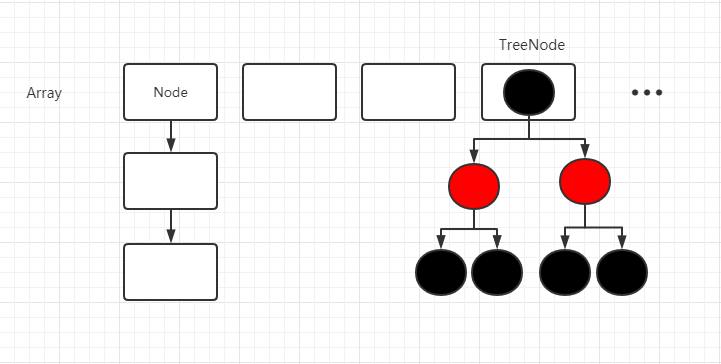### 1.HashMap的存取原理

put方法

``````    public V put(K key, V value) {
return putVal(hash(key), key, value, false, true);
}
``````
``````final V putVal(int hash, K key, V value, boolean onlyIfAbsent,
boolean evict) {
Node<K,V>[] tab; Node<K,V> p; int n, i;
// 当前哈希表为空，则初始化
if ((tab = table) == null || (n = tab.length) == 0)
// 直接调用resize方法，返回长度，默认16
n = (tab = resize()).length;
// index的计算方法 (n - 1) & hash n是tab的Leagth
// 没有hash碰撞，则直接挂在tab[index]下
if ((p = tab[i = (n - 1) & hash]) == null)
tab[i] = newNode(hash, key, value, null);
else {
Node<K,V> e; K k;
// 哈希值相等，key也equals相等，覆盖value
if (p.hash == hash &&
((k = p.key) == key || (key != null && key.equals(k))))
e = p;
else if (p instanceof TreeNode)
e = ((TreeNode<K,V>)p).putTreeVal(this, tab, hash, key, value);
else {
// 遍历链表插入
for (int binCount = 0; ; ++binCount) {
if ((e = p.next) == null) {
p.next = newNode(hash, key, value, null);
// 链表节点数量 >= 8 转化为红黑树
if (binCount >= TREEIFY_THRESHOLD - 1) // -1 for 1st
treeifyBin(tab, hash);
break;
}
// 节点覆盖
if (e.hash == hash &&
((k = e.key) == key || (key != null && key.equals(k))))
break;
p = e;
}
}
// e不是空，有需要覆盖的节点
if (e != null) { // existing mapping for key
V oldValue = e.value;
if (!onlyIfAbsent || oldValue == null)
e.value = value;
// 空函数 Callbacks to allow LinkedHashMap post-actions
afterNodeAccess(e);
return oldValue;
}
}
// 插入新节点完毕，修改modCount
++modCount;

// 更新size 判断是否需要扩容
if (++size > threshold)
resize();
// 空函数 Callbacks to allow LinkedHashMap post-actions
afterNodeInsertion(evict);
return null;
}``````

get方法

``````    public V get(Object key) {
Node<K,V> e;
return (e = getNode(hash(key), key)) == null ? null : e.value;
}

static final int hash(Object key) {
int h;
return (key == null) ? 0 : (h = key.hashCode()) ^ (h >>> 16);
}``````
`````` // 传入扰动后的哈希值 和 key
final Node<K,V> getNode(int hash, Object key) {
Node<K,V>[] tab; Node<K,V> first, e; int n; K k;
if ((tab = table) != null && (n = tab.length) > 0 &&
(first = tab[(n - 1) & hash]) != null) {
// 链表头节点判断
if (first.hash == hash && // always check first node
((k = first.key) == key || (key != null && key.equals(k))))
return first;
if ((e = first.next) != null) {
if (first instanceof TreeNode)
return ((TreeNode<K,V>)first).getTreeNode(hash, key);
do {
if (e.hash == hash &&
((k = e.key) == key || (key != null && key.equals(k))))
return e;
} while ((e = e.next) != null);
}
}``````

### 2.resize，扩容，以及重新hash(rehash)

HashMap的默认负载因子是0.75f，所以当HashMap数组的长度大于容量＊0.75的时候，会对HashMap进行resize处理。

resize后是一定需要进行rehash的，因为index的计算与数组的长度相关，长度扩大之后，hash计算出来的值自然就发生了变化，这个会在下面hash计算展开详细描述。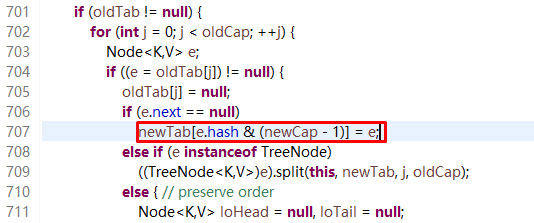### 3.Java7的头插法与Java8的尾插法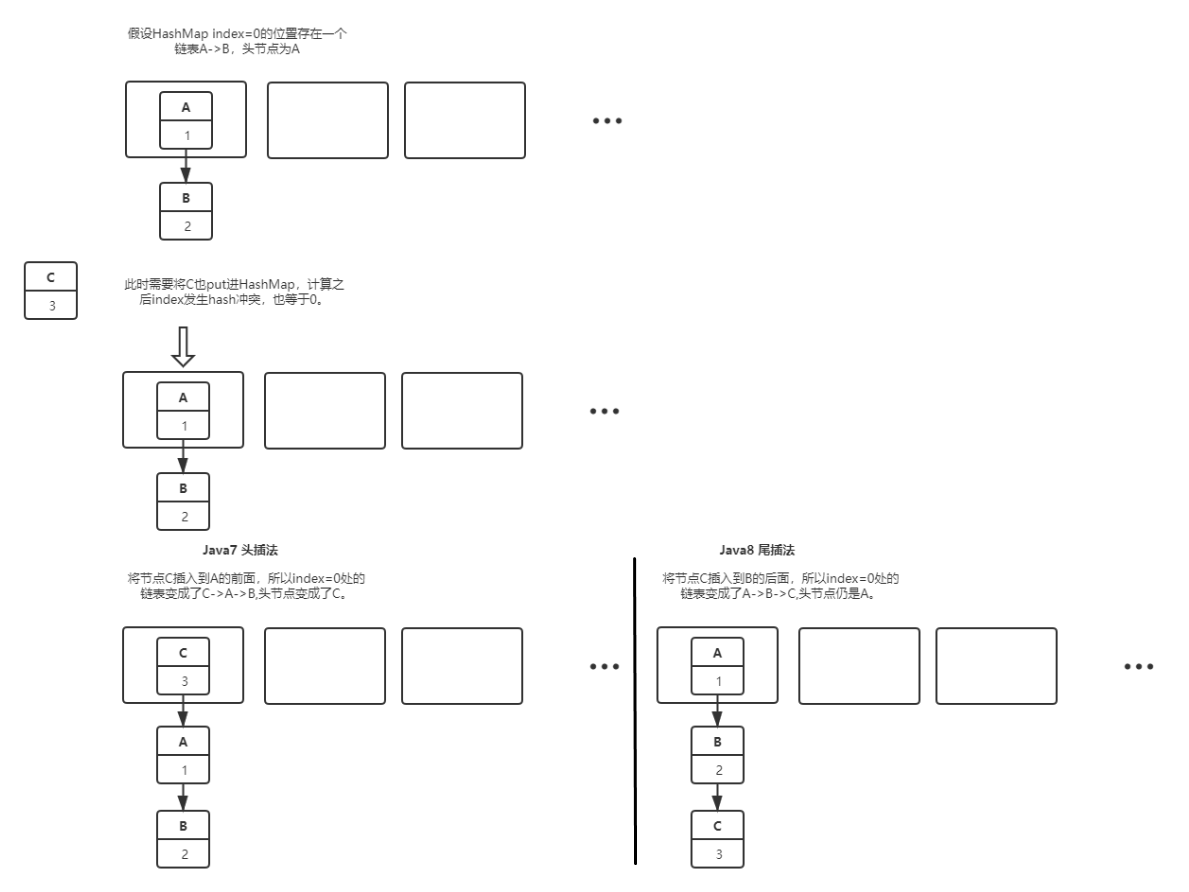## 二，HashMap的主要参数都有哪些？

``````/**
* The default initial capacity - MUST be a power of two.
* 初始化容量必须是2的次方
*/
// 默认初始化容量
static final int DEFAULT_INITIAL_CAPACITY = 1 << 4; // aka 16

// 最大容量
static final int MAXIMUM_CAPACITY = 1 << 30;

// 默认负载因子
static final float DEFAULT_LOAD_FACTOR = 0.75f;

// 链表转红黑树阈值
static final int TREEIFY_THRESHOLD = 8;

// 红黑树转链表阈值
static final int UNTREEIFY_THRESHOLD = 6;

// 树形化最小容量
static final int MIN_TREEIFY_CAPACITY = 64;``````

### 1.初始化容量为什么是16，为什么都要是2的次方？

2的倍数为什么不行？

2的幂数经过Length-1之后，二进制时二进制位全为1，这样计算的index等同于HashCode的后几位的值。

### 2.默认负载因子为什是0.75？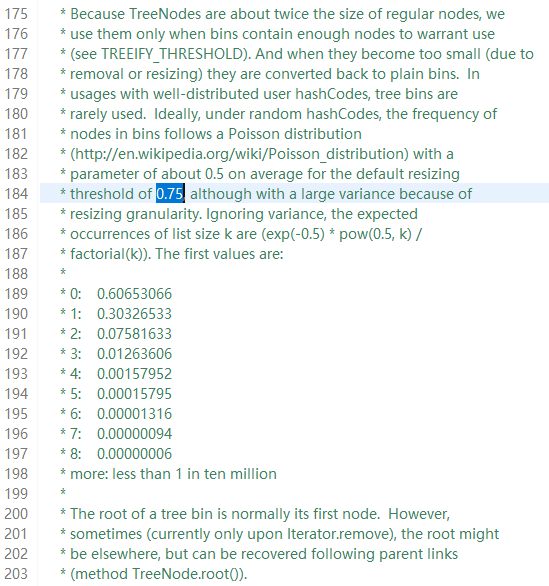0.5的话，虽然可以减少查询时间成本，但是空间的利用率过低，也就意味着会提高rehash操作的次数。

## 三，HashMap中Hash的计算规则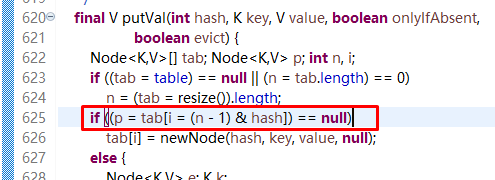### 1.HashMap是怎么处理hash碰撞的？

Hash碰撞或者Hash冲突，指的是计算hash之后所得的Index一致，即发生了hash碰撞，可以看下上面提到的头插法与尾插法，其实就是发生hash碰撞后如何添加数据的。

Java7中的解决办法是链表，Java8变成了链表+红黑树。

### 2.为什么重写equals方法时需要重写hashCode方法？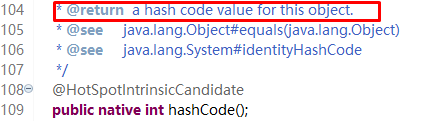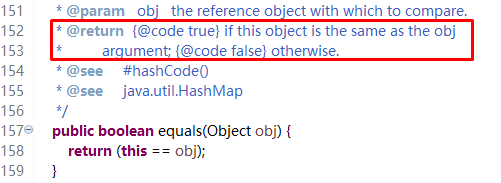## 四，HashMap是否是线程安全

### 1.为啥会线程不安全？

Java7头插法并发情况下出现环形链表，transfer方法

``````void transfer(Entry[] newTable, boolean rehash) {
int newCapacity = newTable.length;
for (Entry<K,V> e : table) {
while(null != e) {
Entry<K,V> next = e.next;
if (rehash) {
e.hash = null == e.key ? 0 : hash(e.key);
}
int i = indexFor(e.hash, newCapacity);
e.next = newTable[i];
newTable[i] = e;
e = next;
}
}
}``````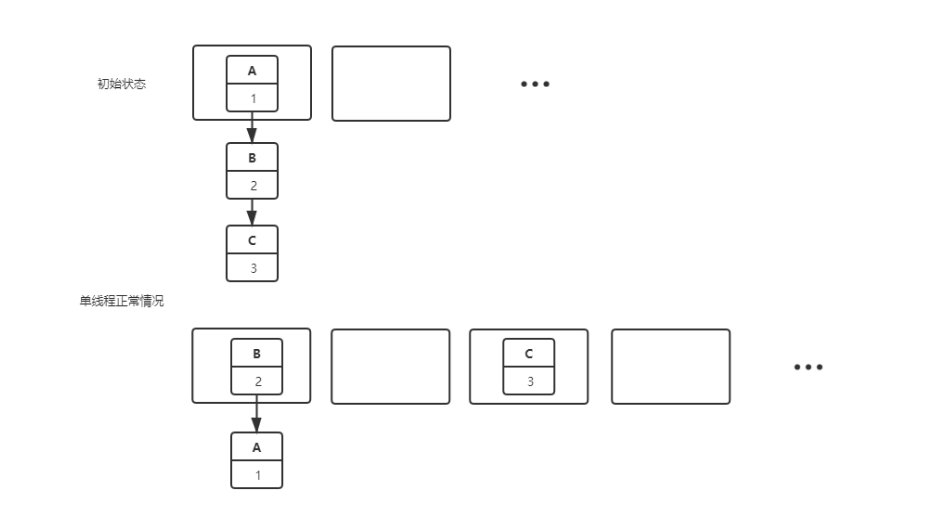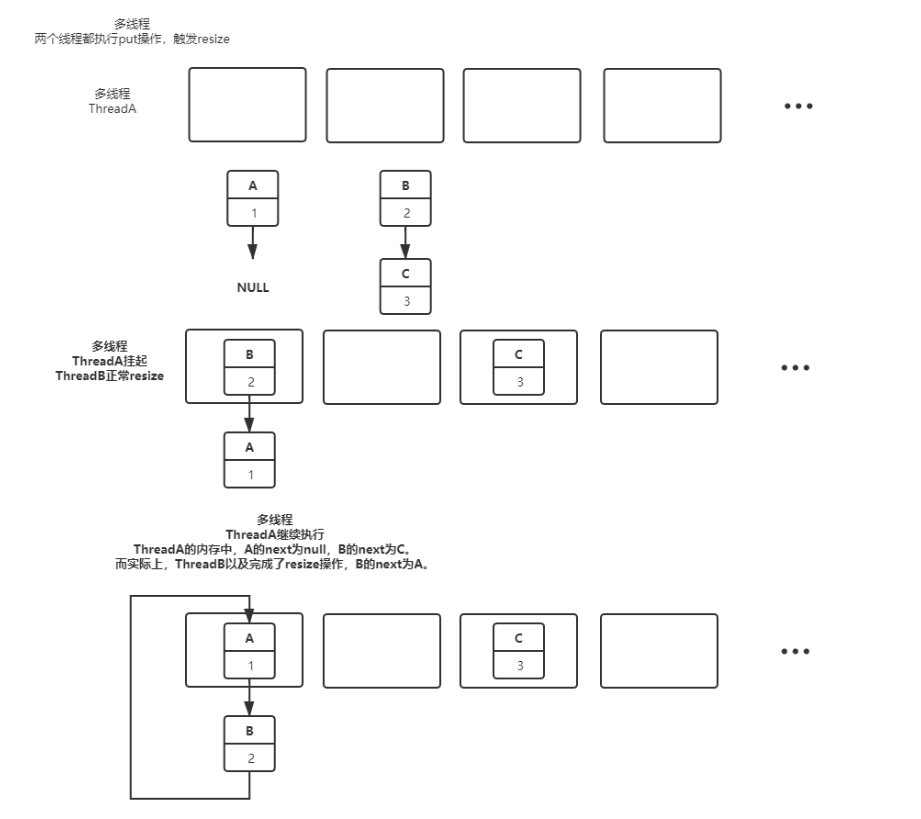Thread A 下图625行判断了tab[index] 为null，可以插入，但是还没有插入时，没有时间片而暂时挂起，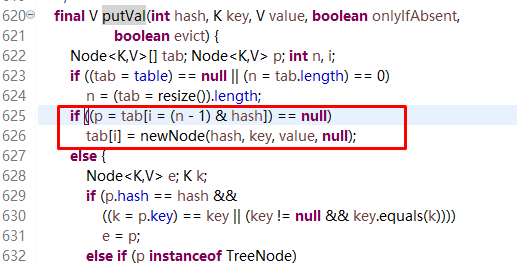### 2.有什么线程安全的类可以替代？

a.Hashtable,对所有方法加锁（synchronized）,所有线程锁的都是当前对象，锁的粒度太大。

b.ConcurrentHashMap，get时没有锁，put时有锁，锁的粒度比较小。

c.Collection.SynchronizedMap，锁的是同一个对象，每次锁的都是当前整张表，锁的粒度太大。

## 五，HashMap的红黑树操作### 低价透明### 金牌服务### 信息保密